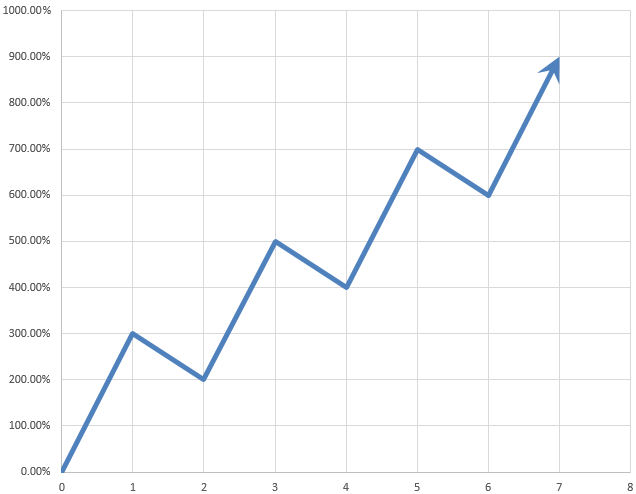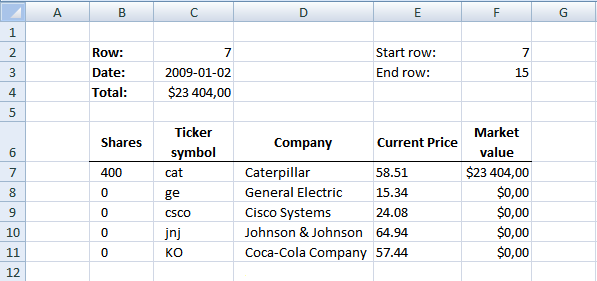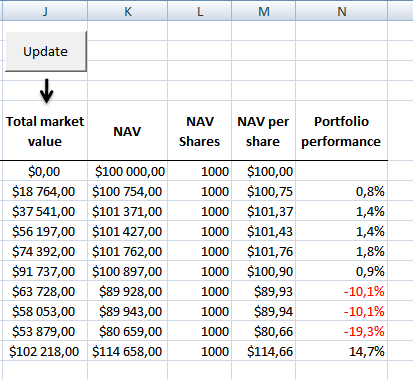Author: Oscar Cronquist Article last updated on February 19, 2018### Introduction

In this post I am creating a spreadsheet that will calculate stock portfolio performance. To do this I am calculating net asset value (NAV).

Net asset value is the value of each stock and the account balance summed, calculated each day as the stock prices fluctuate.

When a deposition or withdrawal is made you add or subtract NAV units to your stock portfolio. More about deposits, withdrawals and NAV units in an upcoming post.

All other transactions affects your stock portfolio performance and is added or subtracted from your account balance.

• Stock prices
• Dividends
• Commissions
• Interest payed or earned

In this excel tutorial I will only buy or sell stocks and calculate each stock's market value (column J) each day. Dates are in column A.

### Transactions sheet

Here is the "Transactions" sheet. It contains some random stock data. Here I calculate stock portfolio market value each day.Formula in H7:

=(F7="BUY")*(-(D7*E7+G7))+(F7="SELL")*((D7*E7-G7))+(F7="Dividend")*(E7*D7)+(OR(F7="Withdrawal", F7="Deposit"))*E7 + ENTER copied down as far as needed

Formula in I7:

=H7+I6 + ENTER copied down as far as needed.

### NAV calculation sheet

In this sheet I calculate stock portfolio market value for each day. Excel queries yahoo and copies close price for each stock into E7 and down. Market value is calculated in F7 and down  and the total is calculated in C4. The total is then copied into sheet "Transactions".Formula in F3:

=COUNTA(Transactions!A:A)+4 + ENTER

Formula in C3:

=INDEX(Transactions!\$A\$1:\$A\$1000,'NAV calc'!C2) + ENTER

Formula in C4:

=SUM(F7:F1000) + ENTER

Formula in B7:

=IF(C7="", "", SUMPRODUCT((Transactions!\$F\$6:\$F\$1000="BUY")* (ROW(Transactions!\$A\$6:\$A\$1000)<='NAV calc'!\$C\$2)*(C7=Transactions!\$C\$6:\$C\$1000)* Transactions!\$D\$6:\$D\$1000)- SUMPRODUCT((Transactions!\$F\$6:\$F\$1000="SELL")* (ROW(Transactions!\$A\$6:\$A\$1000)<='NAV calc'!\$C\$2)* (C7=Transactions!\$C\$6:\$C\$1000)*Transactions!\$D\$6:\$D\$1000)) + ENTER.

Copy cell and paste down as far as needed.

Formula in C7:

=IF(ISNA(INDEX(Transactions!\$C\$7:\$C\$1000, MATCH('NAV calc'!D7, Transactions!\$B\$7:\$B\$1000, 0))), "", INDEX(Transactions!\$C\$7:\$C\$1000, MATCH('NAV calc'!D7, Transactions!\$B\$7:\$B\$1000, 0))) + ENTER

Copy cell and paste down as far as needed.

Formula in D7:

=IF(ISNA(INDEX(Transactions!\$B\$7:\$B\$101, MATCH(0, COUNTIF(\$D\$6:D6, Transactions!\$B\$7:\$B\$101), 0))), "", INDEX(Transactions!\$B\$7:\$B\$101, MATCH(0, COUNTIF(\$D\$6:D6, Transactions!\$B\$7:\$B\$101), 0))) + CTRL + SHIFT + ENTER

Copy cell and paste down as far as needed.

Formula in F7:

=IF(B7=0, 0, IF(C7="", "", B7*E7)) + ENTER.

Copy cell and paste down as far as needed.

### Web Query sheet

I created a web query in cell A5 on sheet "Web query".

http://table.finance.yahoo.com/table.csv?a=["m","m"]&b=["d","d"]&c=["y","y"]&d=["m","m"]&e=["d","d"]&f=["y","y"]&s=["ticker", "ticker"]&y=0&g=d&ignore=.csv

Parameters

y = cell P2

m = cell P3

d = cell P4

ticker = cell P5

Formula in P1:

='NAV calc'!C3 + ENTER

Formula in P2:

=YEAR(P1) + ENTER

Formula in P3:

=MONTH(P1)-1 + ENTER

Formula in P4:

=DAY(P1) + ENTER

### VBA code:

```Sub Calc_nav()

Application.ScreenUpdating = False

Dim rng As Range

Dim price As Range

Dim dest As Range

Dim mnt As Range

Dim i As Integer

Dim qryTblStocks  As QueryTable

'Iterate rows

i = Worksheets("Nav calc").Range("F2")

Set mnt = Worksheets("Transactions").Range("J7")

Do While i <= Worksheets("Nav calc").Range("F3")

Worksheets("NAV calc").Range("C2").Value = i

'Iterate stock symbols

Set rng = Worksheets("NAV calc").Range("C7")

Set price = Worksheets("NAV calc").Range("E7")

Set dest = Worksheets("Web query").Range("P5")

Do While rng <> ""

dest.Value = rng.Value

'Refresh web query

Set qryTblStocks = ThisWorkbook.Worksheets("Web query").QueryTables(1)

With qryTblStocks
.Refresh BackgroundQuery:=False
End With

'Text to columns

Sheets("Web query").Select
Range("A5:A6").Select

Selection.Texttocolumns Destination:=Range("B5"), DataType:=xlDelimited, _
TextQualifier:=xlDoubleQuote, ConsecutiveDelimiter:=False, Tab:=False, _
Semicolon:=False, Comma:=True, Space:=False, Other:=False, FieldInfo _
:=Array(Array(1, 1), Array(2, 1), Array(3, 1), Array(4, 1), Array(5, 1), _
Array(6, 1), Array(7, 1)), TrailingMinusNumbers:=True

'Copy close value Web query F6 to NAV calc E7

price.Value = Worksheets("Web query").Range("F6")

Sheets("Web query").Select
Range("A5:A6").Select
Selection.ClearContents

'Iterate to next cells

Set rng = rng.Offset(1, 0)

Set price = price.Offset(1, 0)

Loop

mnt.Value = Worksheets("NAV calc").Range("C4")

Application.ScreenUpdating = True

Sheets("Transactions").Select

Application.ScreenUpdating = False

Set mnt = mnt.Offset(1, 0)

i = i + 1

Loop

End Sub```

### How to use this spreadsheet

Click "update" button on transactions sheet. Watch market values being calculated in column J.

Here is a picture of transactions sheet when NAV have been calculated.### Final notes

Make sure Transactions sheet is sorted by date.

### Future improvements

Instead of making multiple web queries to yahoo, a better strategy would be to identify the maximum date range and then do one query for each stock in portfolio. This would also speed things up considerably.